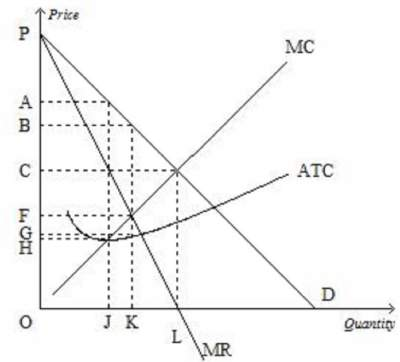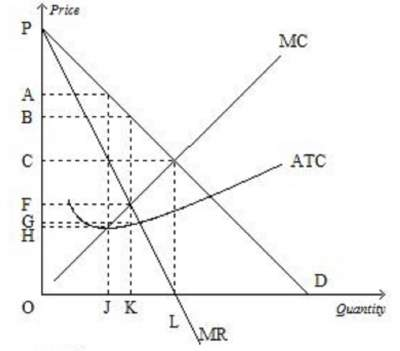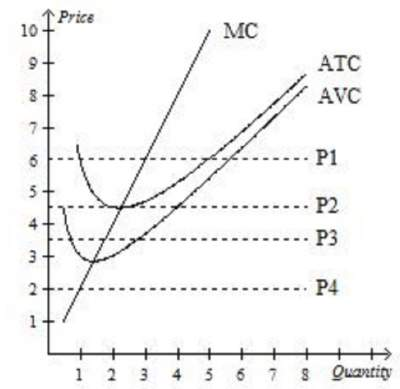# Practice Test on Monopoly: Solutions

Question 1: Which of the following statements is correct? .....
1. A competitive firm is a price maker, whereas a monopolist is a price taker.
2. Both a competitive firm and a monopolist are price makers.
3. A competitive firm is a price taker, whereas a monopolist is a price maker.
4. Both a competitive firm and a monopolist are price takers.
5. 1 and 2

Question 2: Which of the following is not a characteristic of a monopoly? ......
2. barriers to entry.
3. one seller.
4. a product without close substitutes.
5. 2 and 3

Question 3: Which of the following is not a characteristic of a competitive market?
1. Free entry is limited.
2. Each firm chooses an output level that maximizes profits.
3. Each firm sells a virtually identical product.
4. Buyers and sellers are price takers.

Question 4: Which of the following is an example of a barrier to entry? ........
1. hiring marketing experts to increase consumers' brand loyalty.
2. pricing above marginal cost.
3. selling the same good at different prices to different customers.
4. bundling related products to increase total sales.

Question 5: In a competitive market, the actions of any single buyer or seller will ...............
1. Teresa charges a higher price than his competitors for his house-cleaning services.
2. Mary offers free counseling services on Sunday afternoons as a form of advertising. .
3. Lisa obtains a copyright for the new financial application that she invented .
4. Joe charges a lower price than his competitors for his airport pickup services.

Question 6: What price will the monopolist charge?1. H
2. G
3. F
4. D
5. C
6. B
7. A

Question 7: How much output will the monopolist produce?1. H
2. G
3. F
4. D
5. C
6. B
7. A
8. L
9. K
10. J
11. O

Question 8: A profit-maximizing monopoly's total revenue is equal to .....1. (P4 − P3) × Q2
2. (P4 − P2) × Q2.
3. P3 × Q4.
4. P4 × Q2
5. none of the above

Question 9: A profit-maximizing monopoly's total revenue is equal to .....1. (P4 − P3) × Q4
2. (P4 − P2) × Q2.
3. (P5 − P0) × Q1
4. P4 × Q2
5. (P4 − P1) × Q3

Question 10: Which of the following is a characteristic of monopolistic competition?
1. patents.
3. free entry.
4. ownership of a key resource by a single firm.
5. none of the above

Assume a profit-maximizing monopolist charges a price of \$20.
The intersection of the marginal revenue and marginal cost curves occurs where output is 15 units and marginal cost is \$13.
Average total cost for 15 units of output is \$11.
Question 11: What is the monopolist's profit?

1. \$300
2. \$165.
3. \$135
4. \$100.